# Institut für Thermodynamik der Luft- und Raumfahrt - Universität StuttgartMechanical Engineering - The University of Texas at Austin# output files

The out.txt file contains the primary results of the integration, and the print interval is controlled by the kspace variable. Note the first print location for external flows is always at integration step 5 (intg=5) rather than at x=xstart (which would be intg=0) and the last print location is at x=xend. Intermediate output occurs at integration steps that are multiples of kspace.

The ftn.txt files are created whenever the input data flag k5 is set >0, and they are formatted for easy use with plotting packages. These files include:

The ftn74.txt file contains profiles of the dependent variables, printed using three options:

out.txt - Standard Output when kout=2 - This file can be used with all kgeom external flows.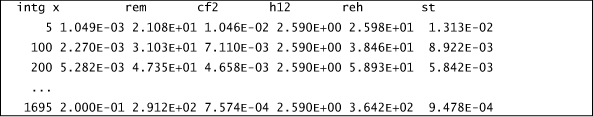where the following TEXSTAN variables translate into textbook variables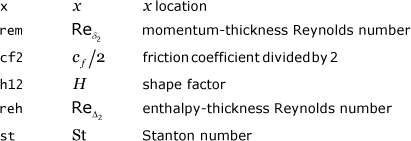out.txt - Benchmark Output when kout=8 - This file has been designed for used only with select datasets of the kgeom=1 class of external flow.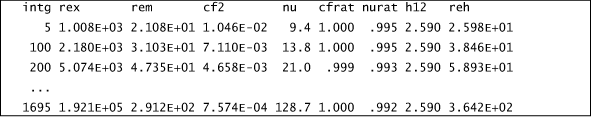where the following TEXSTAN variables translate into textbook variables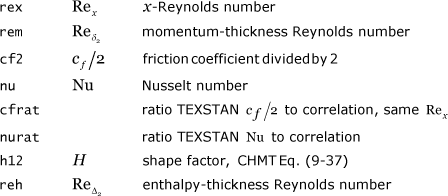ftn84.txt - Boundary Layer Profile Information - This file prints boundary layer profile information for all external flows.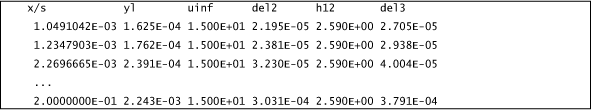where the following TEXSTAN variables translate into textbook variables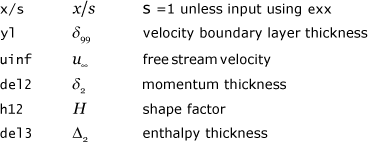and

ftn85.txt - Reynolds Numbers, Friction, and Heat Transfer - This file prints Reynolds numbers, friction and heat transfer distributions for all external flows.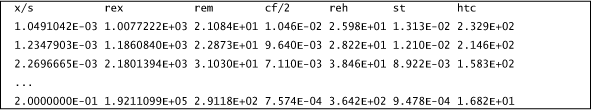where the following TEXSTAN variables translate into textbook variablesftn86.txt - Surface Heat Flux - This file prints the surface heat flux distribution when heat transfer is being calculated.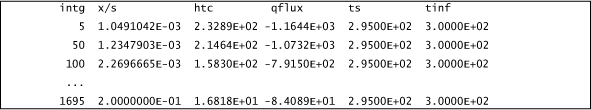where the following TEXSTAN variables translate into textbook variablesftn87.txt - Stanton Number - This file prints the Stanton number distribution when heat transfer is being calculated.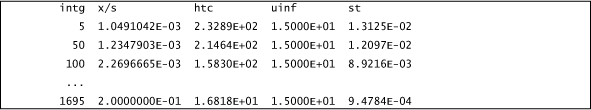where the following TEXSTAN variables translate into textbook variablesftn88.txt - Surface Mass Flux - This file prints the surface mass flux distribution when mass transfer is being calculated.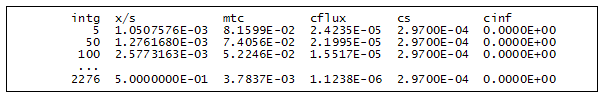where the following TEXSTAN variables translate into textbook variablesftn89.txt - Mass Transfer Stanton Number - This file prints the mass transfer Stanton number distribution when mass transfer is being calculated.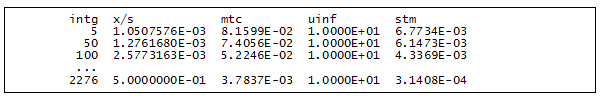where the following TEXSTAN variables translate into textbook variablesftn74.txt - Standard Profiles - at all x(m) locations when k10=11 - This first option prints profiles at xstart and at xend and at all x(m) locations between xstart and xend. The kspace variable is ignored. The user can further control where profiles are printed by adding additional x(m) locations and their corresponding boundary conditions. For example, in s10.dat.txt the variable nxbc=5 and there are 5 x(m) locations, although technically only two boundary condition locations are needed for a dataset that has a constant free stream velocity and a constant surface temperature or heat flux boundary condition.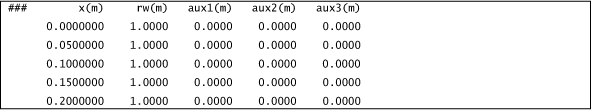Here is a sample of the profile information in file ftn74.txt at the location x(m=5)=xend.where the following TEXSTAN variables translate into textbook variablesftn74.txt - Benchmark Profiles - flat plate - at all x(m) locations when k10=10 - This second option for printing profiles is similar to the first option, but it has been designed for use only with select datasets of the kgeom=1 class of external flow. The profiles have been normalized using variables that are consistent with whether the benchmark test case is laminar flow or turbulent flow.
laminar flow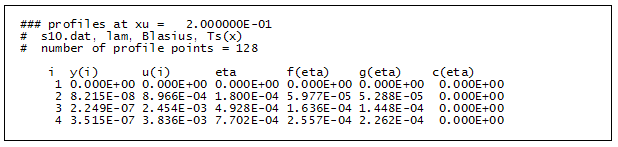where the following TEXSTAN variables translate into textbook variablesturbulent flowwhere the following TEXSTAN variables translate into textbook variables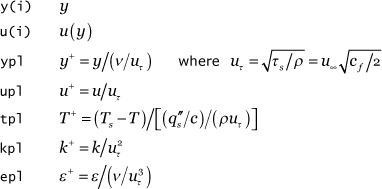ftn74.txt - Single Set of Profiles - at a specified location using the k10 variable - This third option for printing profiles is to set k10 =21 (or) =22 (or) =23 to cause profiles of all the dependent variables to be printed at a single location. By setting k10=21, the profiles will be printed when the x-Reynolds number equals (or exceeds) a value defined by the input variable bxx. For k10=22, the bxx value will be interpreted as a momentum-thickness Reynolds number, and for k10=23, the bxx value will be interpreted as an enthalpy-thickness Reynolds number. This set of profiles is written to files ftn73.txt and ftn74.txt. The files are mostly identical, but the file ftn73.txt is formatted in a special way for use with kstart=0 to restart TEXSTAN, and this format is discussed in the external flows: initial profiles part of this section. For plotting and analysis purposes, the file ftn74.txt is more meaningful. The output structure of this profile is identical to that described above for k10=11 and not reprinted here.

website updated Sept 2010   © 1996-2010 Michael E. Crawford - all rights reserved - website validated for CSS 2.1 and XHTML 1.0 strict at www.w3.org